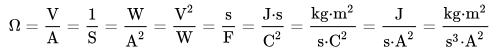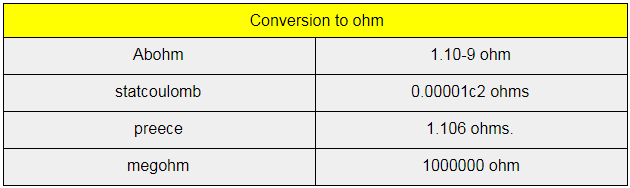# Unit of Resistance

Resistance is a term that students come across often when they study about electric currents. While electric current has two factors namely conductance and resistance we will have a quick look at the latter here in this page. Talking about resistance, it is basically defined as the obstruction that a substance causes to the flow of electric current. Resistance is represented by the letter R and has the dimension length squared mass per time cubed electric current squared. Below we will look at the unit of resistance and other details.

## SI Unit of Resistance

The SI unit of resistance is ohm, which is defined as a volt per ampere. The name has been kept in remembrance of German physicist George Simon Ohm. The unit is sometimes written out as a word or symbolized by the uppercase Greek letter omega. Ohm has the following formula;in which the following units also appear: ampere (A), Siemens (S), volt (V), watt (W), second (s), metre (m), joule (J), farad (F), kilogram (kg), and Coulomb (C).

There is something called specific resistance or resistivity which is measured by the unit ohm-meter or ohm-m.

### Popular Resistance Units

Some of the popular units of resistance include Abohm, megohm, statohm, preece, and planck-impedance. Check out the conversion below.Stay tuned with BYJU’S for more such interesting articles. Also, register to “BYJU’S – The Learning App” for loads of interactive, engaging Physics-related videos and an unlimited academic assist.

Related articles:

Test your Knowledge on unit of resistance